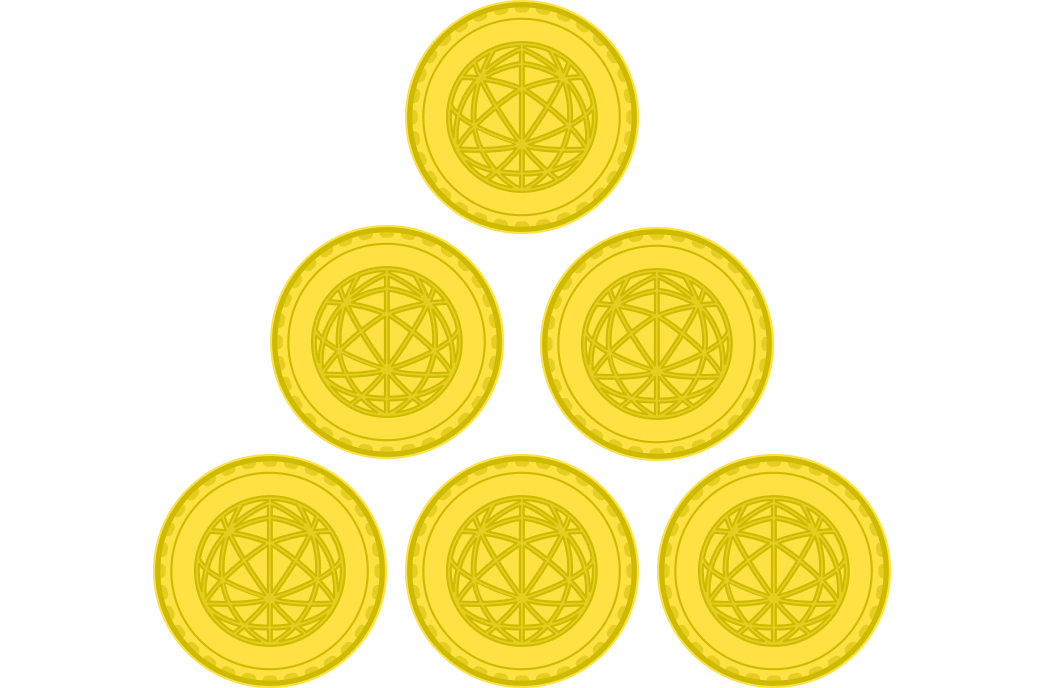# Upside-Down Triangles

Probability Level 3To turn a three-story pyramid of coins $($with $1 + 2 + 3 = 6$ coins$)$ upside down, we only need to move 2 coins: moving the bottom left and bottom right coins next to the top coin.

Now, what if there are $1 + 2 + 3 +\cdots+100=5050$ coins instead? What is the minimum number of coins we have to move in order to turn this huge, 100-story pyramid upside down?

Bonus: Generalize this for a pyramid of $1+2+3+\cdots+n$ coins.

×